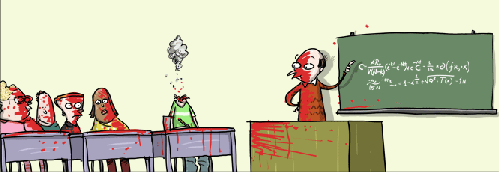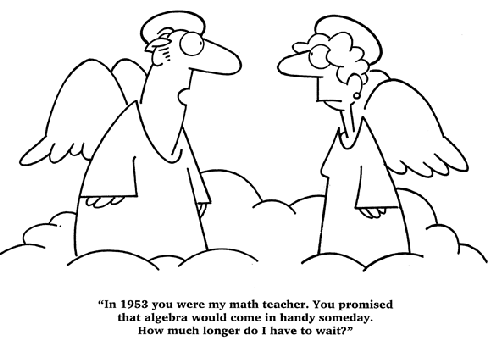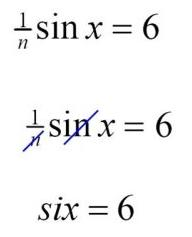# Jokes | Mathematics Centre## Jokes

You will find many mathematical games [ here ].

Q: What does an employed mathematician say to the unemployed mathematician?
A: "Do you want fries with that?"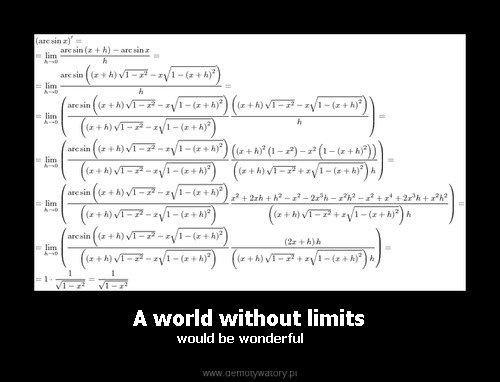- As usual: between e and pi.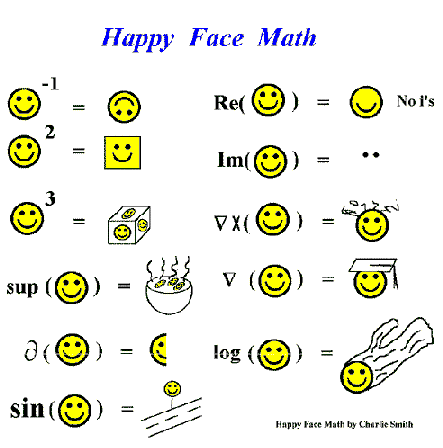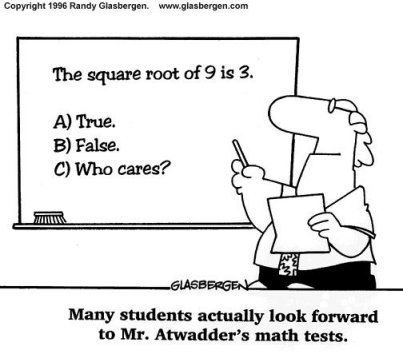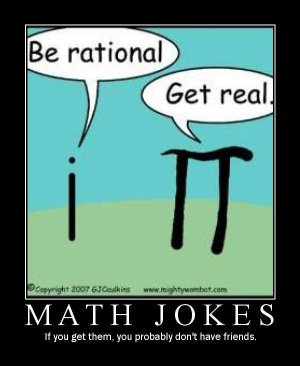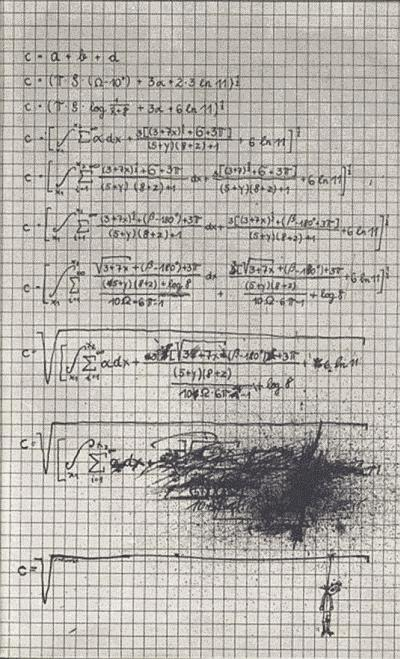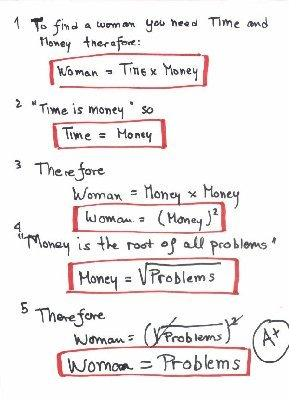Q: What is a derivative of a party?
A: It's the amount of alcohol that you can buy when you sell the empty bottles after the party.
Q: And when can you say that the party was really good?
A: When the second derivative is poistive as well.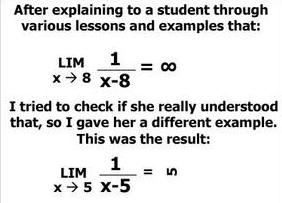The Local Genius Theorem: For every mathematician there exists a surrounding in which he is the most ingenious one.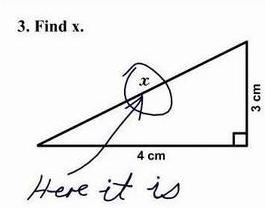An infinite number of mathematicians walk into a bar. The first mathematician asks for one beer. The second mathematician asks for 0.5 beer, the next one asks for 1/4 beer and so on. But the bar-tender just smiles and pours them two beers...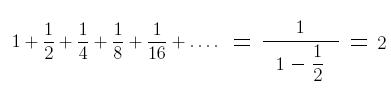Mathematicians never die - they only lose some of their functions.

- What does the zero say to the eight?
- Nice belt!